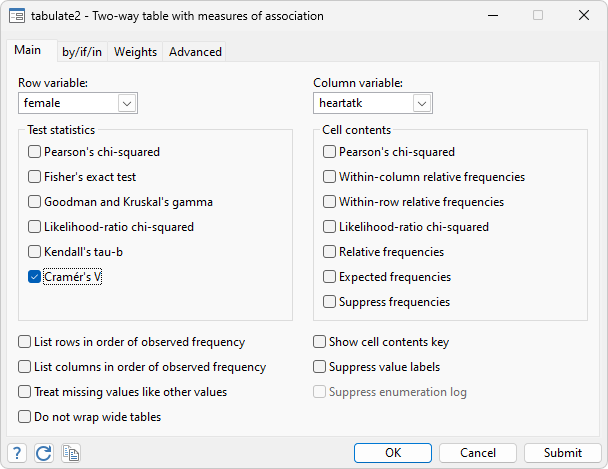Summaries

Arithmetic, geometric, and harmonic means, and corresponding confidence intervals

Medians and percentiles

Measurement of central tendency

Skewness and kurtosis

Confidence intervals

Means

Normal

Exact Poisson

Proportions

Exact binomial (Clopper–Pearson)

Wald

Wilson

Jeffreys

Agresti

Variance

Normal

Bonett

Ratios, proportions, and totals

Confidence intervals

Standard errors

Over groups

Robust and cluster–robust SEs

Intragroup tests

Tables and tabulations

1-, 2-, n-way

Pearson’s chi-squared

Likelihood-ratio chi-squared

Cramér’s V

Fisher’s exact (2 x 2 and r x c)

Goodman and Kruskal’s gamma

Kendall’s tau

Cell statistics

Expected count

Contribution to Pearson chi-squared

Contribution to likelihood-ratio chi-squaredTables of summary statistics

Over groups and/or variables

Statistics may include mean, count, sum, min,max, range, standard deviation, variance, variation coefficient, standard error of mean, skewness, kurtosis, median, percentiles, and interquartile range

Creation of datasets of summary statistics

Statistics by group or subgroup of observations

Statistics: mean, standard deviation, count, min, max, median, percentiles, and interquartile range

Pairwise comparisons of means

Pairwise differences in means with tests and confidence intervals

Multiple-comparison adjustments: Bonferroni, Šidák, Scheffé, Tukey HSD, Duncan, and Student–Newman–Keuls adjustments

Group comparisons that are significant

Balanced and unbalanced data

t tests

One sample

Two independent samples

Two paired samples

Based on summary data (ttesti)

z tests

One sample

Two independent samples

Two paired samples

Support for clustered data

Based on summary data (ztesti)

Tests of proportions

One sample

Two sample

Support for clustered data

Based on summary data (prtesti)

Effect sizes

Comparison of means

Cohen’s d

Hedge’s g

Glass’s Δ

Point/biserial correlation

Confidence intervals

Variance explained by regression and ANOVA

Eta-squared—η2

Omega-squared—ω2

Confidence intervals

Watch A tour of effect sizes.

Other common tests

Binomial (one-sample) test

Test of proportions (two sample)

Support for clustered data

Bartlett’s test

Chi-squared (one-sample) variance test

Variance ratio (two-sample) F test

Correlations

Pearson simple and partial

Semipartial

Spearman’s rank order

Kendall’s rank order

Intracluster

Cronbach’s alpha

Tetrachoric

Canonical (multivariate) correlations

Intraclass

Factor variables

Automatically create indicators based on categorical variables

Form interactions among discrete and continuous variables

Include polynomial terms

Perform contrasts of categories/levels

Watch Introduction to Factor Variables in Stata tutorials